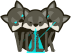Vector Optimized Library of Kernels  3.0.0 Architecture-tuned implementations of math kernelsOverview

Selects maximum value from each entry between bVector and aVector and store their results in the cVector.

c[i] = max(a[i], b[i])

Dispatcher Prototype

void volk_32f_x2_max_32f(float* cVector, const float* aVector, const float* bVector,
unsigned int num_points)

Inputs

• aVector: First input vector.
• bVector: Second input vector.
• num_points: The number of values in both input vectors.

Outputs

• cVector: The output vector.

Example

int N = 10;
unsigned int alignment = volk_get_alignment();
float* increasing = (float*)volk_malloc(sizeof(float)*N, alignment);
float* decreasing = (float*)volk_malloc(sizeof(float)*N, alignment);
float* out = (float*)volk_malloc(sizeof(float)*N, alignment);
for(unsigned int ii = 0; ii < N; ++ii){
increasing[ii] = (float)ii;
decreasing[ii] = 10.f - (float)ii;
}
volk_32f_x2_max_32f(out, increasing, decreasing, N);
for(unsigned int ii = 0; ii < N; ++ii){
printf("out[%u] = %1.2f\n", ii, out[ii]);
}
volk_free(increasing);
volk_free(decreasing);
volk_free(out);
size_t volk_get_alignment(void)
Get the machine alignment in bytes.
Definition: volk.tmpl.c:90
__VOLK_DECL_BEGIN VOLK_API void * volk_malloc(size_t size, size_t alignment)
Allocate size bytes of data aligned to alignment.
Definition: volk_malloc.c:38
VOLK_API void volk_free(void *aptr)
Free's memory allocated by volk_malloc.
Definition: volk_malloc.c:80